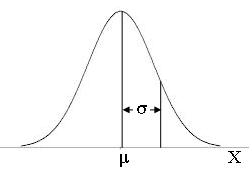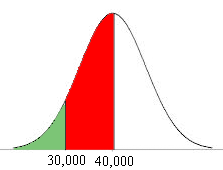SEARCH HOMEMath Central Quandaries & QueriesQuestion from Melanie, a parent: This is the question: The lifetime of a certain type of car tire are normally distributed. The mean lifetime of a car tire is 40,000 miles with a standard deviation of 5,000 miles. Consider a sample of 10,000 tires. A) How many tires would you expect to last between 35,000 and 45,000 miles? b) How many tires would you expect to last between 30,000 and 40,000 miles? c) How many tires would you expect to last less than 40,000 miles? d)How many tires would you expect to last more than 50,000 miles? e) How many tires would you expect to last less then 25,000 miles? f) What tires would you want on your car and explain your reasoning Here is a portion of the answer we've come up with: 68.3(10,000)=6,830 tires will last between 35,000 and 45,000 miles 95.5(10,000)=9,550 tires will last between 30,000 and 40,000 miles 99.7(10,000)=9,970 tires will last less than 40,000 miles Not at all sure that we've done any of this correctly and not sure how to determine how many tires will last less than 25,000 miles. Any help is appreciated.Hi Melanie,

Here is a sketch of a random variable $X$ which has the normal distribution with mean mu, $(\mu)$ and standard deviation sigma $(\sigma).$The area bounded by the curve and the X-axis is 1 and the curve is symmetric about $X = \mu$ and hence the area under the curve to the left of $X = \mu$ must be 1/2. This area is the probability that $X$ is less than $\mu$ and hence

$Pr(X < \mu) = \frac12 .$

In your example $mu = 40,000$ miles and you would expect half of the tires in the sample to last less than 40,000 miles. Thus the answer to part c) is 5,000 tires.

To answer the remaining parts of this problem you need to convert $X$ to the standard normal distribution and use a standard normal distribution table. I'll do part b) for you and you can do the rest. Here is the diagram I drew.Normal distribution with $\mu = 40,000$ and $\sigma = 5,000.$

The probability that $X$ is between 30,000 and 40,000 miles is the area under the curve between 30,000 and 40,000, the red area in the diagram. To calculate this probability I am going the calculate the area under the curve to the left of 30,000 miles, that area shaded green in the diagram and then

$Pr(30,000 < X < 40,000) = Pr(X < 40,000) - Pr(X < 30,000).$

Since I already know that $Pr(X < 40,000) = \large \frac{1}{2}$ I only need to find $Pr(X < 30,000).$ To do this I need to use the standard normal distribution.

To convert a normal random variable with mean mu and standard distribution to the standard normal random variable $Z$ the conversion is

$Z = \frac{X - \mu}{\sigma}$

which in your case is

$Z = \frac{X - 40,000}{5,000}.$

Hence

$Pr(X < 30,000) = Pr \left( \frac{X - \mu}{\sigma} < \frac{30,000 - 40,000}{5,000} \right)$

or

$Pr(X < 30,000) = Pr\left( Z < -2 \right).$

Again by the symmetry of the normal distribution $Pr(Z < -2) = Pr(Z > 2).$ (If this isn't clear look at a diagram.)

My standard normal table gives probabilities of the form $Pr(0 < Z < a)$ for various values of $a$ and in particular it gives $Pr(0 < Z < 2) = 0.4772$ and hence $Pr(Z > 2) = \frac12 - Pr(0 < Z < 2) = 0.0223.$

Finally

$Pr(30,000 < X < 40,000) = Pr(X < 40,000) - Pr(X < 30,000) = \frac12 - 0.0223 = 0.4772.$

and thus the number of tires expected to last between 30,000 and 40,000 miles is

$10,000 \times 0.4772 = 4,772.$

PennyMath Central is supported by the University of Regina and The Pacific Institute for the Mathematical Sciences.